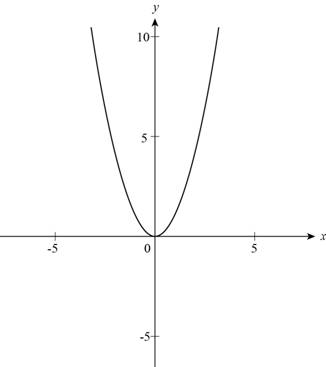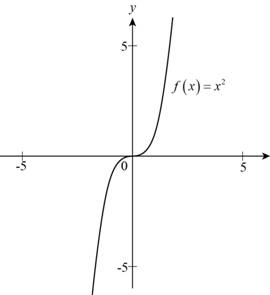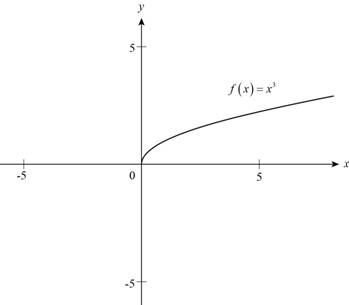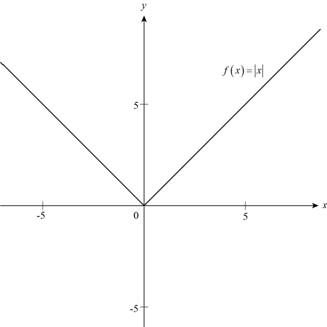# The graph of given function.### Precalculus: Mathematics for Calcu...

6th Edition
Stewart + 5 others
Publisher: Cengage Learning
ISBN: 9780840068071### Precalculus: Mathematics for Calcu...

6th Edition
Stewart + 5 others
Publisher: Cengage Learning
ISBN: 9780840068071

#### Solutions

Chapter 2.2, Problem 4E

(a)

To determine

## To evaluate: The graph of given function.

Expert Solution

The function f(x)=x2 is plotted on the graph which equals the graph IV .

### Explanation of Solution

The given function is,

f(x)=x2 (1)

Substitute 1 for n in given function for first plot,

f(1)=12=1

Substitute 2 for n in given function for second plot,

f(2)=22=4

Substitute 3 for n in given function for third plot,

f(3)=32=9

Substitute 4 for n in given function for fourth plot,

f(4)=42=16

Substitute 5 for n in given function for fifth plot,

f(5)=52=25

The value of table for the above function is:

 x f(x) 1 1 2 4 3 9 4 16 5 25

Use the above value of table, plot the graph as shown in Figure(1),Figure (1)

Where x is plotted in x-axis and f(x) is plotted on y-axis,

Thus the function f(x)=x2 is plotted on the graph which equals the graph IV .

(b)

To determine

### To evaluate: The graph of given function.

Expert Solution

The function f(x)=x3 is plotted on the graph which equals the graph II .

### Explanation of Solution

The given function is f(x)=x3 .

Substitute 1 for n in given function for first plot,

f(1)=13=1

Substitute 2 for n in given function for second plot,

f(2)=23=8

Substitute 3 for n in given function for third plot,

f(3)=33=27

Substitute 4 for n in given function for fourth plot,

f(4)=43=64

Substitute 5 for n in given function for fifth plot,

f(5)=53=125

The value of table for the above function is:

 x f(x) 1 1 2 8 3 27 4 64 5 125

Use the above value of table, plot the graph as shown in Figure (2),Figure (2)

Where x is plotted in x-axis and f(x) is plotted on y-axis,

Thus the function f(x)=x3 is plotted on the graph which equals the graph II .

(c)

To determine

### To evaluate: The graph of given function.

Expert Solution

The function f(x)=x3 is plotted on the graph which equals the graph I .

### Explanation of Solution

The given function is f(x)=x .

Substitute 1 for n in given function for first plot,

f(1)=1=1

Substitute 2 for n in given function for second plot,

f(2)=2=1.414

Substitute 3 for n in given function for third plot,

f(3)=3=1.732

Substitute 4 for n in given function for fourth plot,

f(4)=4=2

Substitute 5 for n in given function for fifth plot,

f(5)=5=2.24

The value of table for the above function is:

 x f(x) 1 1 2 1.414 3 1.732 4 2 5 2.24

Use the above value of table, plot the graph as shown in Figure (3),Figure (3)

Where x is plotted in x-axis and f(x) is plotted on y-axis,

Thus, the function f(x)=x3 is plotted on the graph which equals the graph I .

(d)

To determine

### To evaluate: The graph of given function.

Expert Solution

The function f(x)=|x| is plotted on the graph which equals the graph III .

### Explanation of Solution

The given function is f(x)=|x| .

Substitute 1 for n in given function for first plot,

f(1)=|1|=±1

Substitute 2 for n in given function for second plot,

f(2)=|2|=±2

Substitute 3 for n in given function for third plot,

f(3)=|3|=±3

Substitute 4 for n in given function for fourth plot,

f(4)=|4|=±4

Substitute 5 for n in given function for fifth plot,

f(5)=|5|=±5

The value of table for the above function is:

 x f(x) 1 ±1 2 ±2 3 ±3 4 ±4 5 ±5

Use the above value of table, plot the graph as shown in Figure(4),Figure (4)

Where x is plotted in x-axis and f(x) is plotted on y-axis.

Thus, the function f(x)=|x| is plotted on the graph which equals the graph III .

### Have a homework question?

Subscribe to bartleby learn! Ask subject matter experts 30 homework questions each month. Plus, you’ll have access to millions of step-by-step textbook answers!